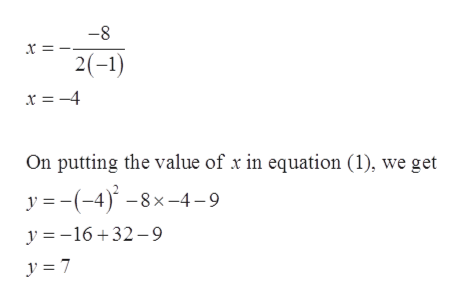# Find the coordinates of the vertex for the parabola defined by the given quadratic function f(x)=-x2-8x-9

Question
6 views

Find the coordinates of the vertex for the parabola defined by the given quadratic function

f(x)=-x2-8x-9

check_circle

Step 1

Consider the given equation of the parabola.

Step 2

The standard quadratic equation form, y = ax2 + bx + c

Step 3

Therefore, the vertex of the ...help_outlineImage Transcriptionclose-8 x 2(-1) x = -4 On putting the value of x in equation (1), we get y (-4)8x-4-9 y 16+32-9 y = 7 fullscreen

### Want to see the full answer?

See Solution

#### Want to see this answer and more?

Solutions are written by subject experts who are available 24/7. Questions are typically answered within 1 hour.*

See Solution
*Response times may vary by subject and question.
Tagged in

### Algebra